×#### Thank you for registering.

One of our academic counsellors will contact you within 1 working day.

Click to Chat

1800-1023-196

+91-120-4616500

CART 0

• 0

MY CART (5)

Use Coupon: CART20 and get 20% off on all online Study Material

ITEM
DETAILS
MRP
DISCOUNT
FINAL PRICE
Total Price: Rs.

There are no items in this cart.
Continue Shopping• Complete JEE Main/Advanced Course and Test Series
• OFFERED PRICE: Rs. 15,900
• View Details

```Solved Examples on Gravitation & Projectile

Question 1:- At what initial speed must the basketball player throw the ball, at 55º above the horizontal, to make the foul shot, as shown in below figure? The basketball rim is 18 in. in diameter. Obtain other data from below figure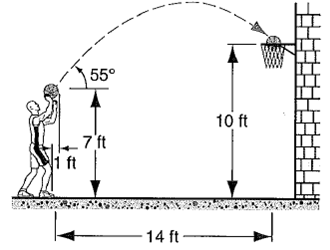Concept:

The figure below shows the basketball player making a foul shot:It can be seen from the figure that the effective horizontal distance to make that shot is

14ft -1ft=13ft whereas the effective vertical distance travelled by the ball is 10 ft - 7 ft = 3 ft

Assume that the angle at which the ball is released with initial velocity v0 is θ such that the horizontal component of initial velocity is v0x = v0 cosθ and the vertical component of initial velocity is v0y = v0 sinθ.

The time taken by the ball to reach the basket can be written in terms of horizontal component of velocity and the effective horizontal distance travelled by the ball as:

t = 13 ft / v0x

Substitute v0x = v0 cosθ,

t = 13 ft / v0 cosθ               …… (1)

Similarly, one can write the time t in terms of the vertical component on initial velocity and the effective vertical distance travelled by the ball as:

3ft = v0yt – ½ gt2

Substitute v0y = v0 sinθ,

3ft = (v0 sinθ)t – ½ gt2

Substitute t = 13 ft/ v0 cosθ  from equation (1),Substitute the value of θ and g to obtain the value of v0.

Solution:

Substitute 55º  for θ and 32.2 ft/s2  for g in equation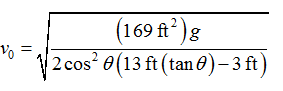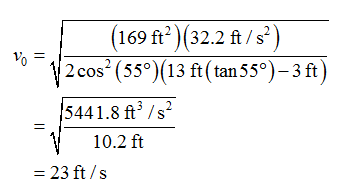Therefore, the magnitude of initial velocity of the ball is 23 ft/s.Question 2:-A spherical hollow is made in a lead sphere of radius R, such that its surface touches the outside surface of the lead sphere and passes through its center (see below figure). The mass of the sphere before hollowing was M. With what force, according to the law of universal gravitation, will the hollowed lead sphere attract a small sphere of mass m, which lies at a distance d from the center of the lead sphere on the straight line connecting the centers of the spheres and of the follow?  [1946, Olympic, Moscow State University]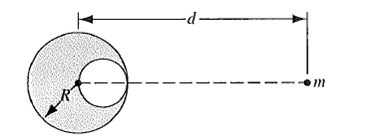Concept:

The force of gravity on the small sphere of mass  due to the lead sphere after it is made hollow is equal to the force of gravity from a solid lead sphere minus the force which would have been contributed by the smaller lead sphere which would have filled the hole.

Solution:

From the Newton’s law of universal gravitation, the force of attraction between the lead sphere and the small sphere is

F1 = GMm/d2

Here, gravitational constant is G, total mass of the lead sphere is M, mass of the small sphere is m and distance between the centers of the spheres is d.

So, we need to know about the size and mass of the lead which was removed to make the hole.

The density of the lead is given by

ρ = M/[(4/3)(πR3)]

The spherical sphere has a radius of R/2.

If the density is constant, then the mass of the hole will be the density times the volume of the hollow sphere. Thus, the mass of the hollow sphere is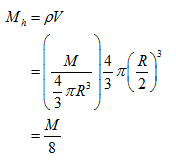The hole is closer to the small sphere.  This means the center of the hole is d-(R/2) away from the small sphere.

Now, the gravitational force between the hollow sphere and the small sphere is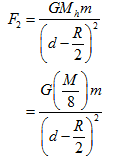The force of attraction with which the lead sphere after it is made hollow and the small sphere is equal to the force of the whole lead sphere before it is made hollow minus the force of the hollow lead sphere. The corresponding force isTherefore, the required force from the lead sphere which attract the small sphere isQuestion 3:-A certain triple-star system consists of two stars, each of mass m, revolving about a central star; mass M, in the same circular orbit. The two stars stay at opposite ends of a diameter of the circular orbit; see below figure. Derive an expression for the period of revolution of the stars; the radius of the orbit is r.Concept:

The gravitational force due to the two stars to the central star is

F1 = GMm/r2 + GMm/r2

= 2 GMm/r2

Here, gravitational constant is G, mass of the central star is M, mass of the orbiting stars is m and radius of the orbit is r.

Also, the gravitational force between the orbiting stars is,

F2 = Gmm/(2r)2

= Gm2/4r2

The net gravitational force acting on the stars is equal to the sum of the gravitational forces acting on the system. It is given as,

Fnet = F1+F2

= 2 GMm/r2 + Gm2/4r2

= Gm/r2 [(2M)+m/4]

The centripetal force that makes the stars to orbit around the central star is

Fc = 4π2mr/T2

Here, period of the stars around the central star is T.

Solution:

The centripetal force is provided by the net gravitational force acting on the system of stars. Thus,

Fnet = Fc

Substitute Gm/r2 [(2M)+m/4] for Fnet  and 4π2mr/T2 for Fc in the above equation.

Fnet = Fc

Gm/r2 [(2M)+m/4] = 4π2mr/T2

So, T = √16π2r3/[G(8M+m)]

Therefore, the expression for the period of revolution of the stars is √16π2r3/[G(8M+m)].
```### Course Features

• 728 Video Lectures
• Revision Notes
• Previous Year Papers
• Mind Map
• Study Planner
• NCERT Solutions
• Discussion Forum
• Test paper with Video Solution